Search

About 10 Search Results Matching Types of Worksheet, Worksheet Section, Generator, Generator Section, Kids Sudoku Puzzle3, Subjects matching Place Value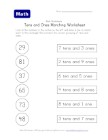Tens and Ones Matching Worksheet - Two of Two

This place value worksheet asks kids to match the ...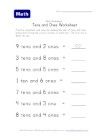Counting Tens and Ones Worksheet

Each question tells kids the number of groups of t...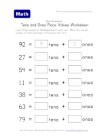Tens and Ones Place Value Worksheet - Two of Two

Break the total number down into its place values ...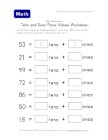Tens and Ones Place Value Worksheet - One of Two

Learn place values by filling in the boxes with th...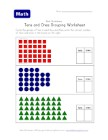Tens and Ones Grouping Worksheet - Two of Two

Circle the group(s) of tens that you can and then ...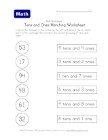Tens and Ones Matching Worksheet - One of Two

Draw a line to match the numbers to the correct gr...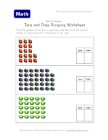Tens and Ones Grouping Worksheet - One of Two

Circle each group of ten and then write the number...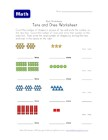Counting Shapes in Tens and Ones

Count the groups and tens and ones - then write th...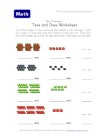Count Fruit in Tens and Ones

Count the number of groups of tens and ones and wr...